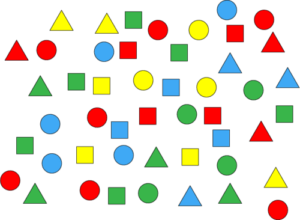# Addition of Whole Numbers with the Sum less than 20 (Primary 1)

Last Updated on July 16, 2020 by Alabi M. S.

MATHEMATICS

SECOND TERM

WEEK 4

PRIMARY 1

THEME – BASIC OPERATIONS

PREVIOUS LESSON – Second Term Scheme of Work and Plan Lesson Notes for MATHEMATICS Week 1 to Week 12 Primary 1 – Revision

TOPIC – ADDITION OF WHOLE NUMBERS WITH THE SUM LESS THAN 20

PERFORMANCE OBJECTIVES

By the end of the lesson, the pupils should have attained the following objectives (cognitive, affective and psychomotor) and should be able to add two whole numbers with the results from 11 to 19 and sum less than 20.

ENTRY BEHAVIOUR

Addition of numbers or objects

INSTRUCTIONAL MATERIALS

The teacher will teach the lesson with the aid of:

1.Oranges

2. Balls

3. Leaves

4. Bottle tops

5. Number beads, etc.

METHOD OF TEACHING – Choose a suitable and appropriate methods for the lessons.

Note – Irrespective of choosing methods of teaching, always introduce an activities that will arouse pupil’s interest or lead them to the lessons.

REFERENCE MATERIALS

1. Scheme of Work

2. 9 – Years Basic Education Curriculum

3. Course Book

4. All Relevant Material

5. Online Information

CONTENT OF THE LESSON

## LESSON ONE – COUNTING TOGETHER

Pupil’s Activities 1 – COUNTING OBJECTSTeacher’s activities – How many circles, triangles and square are on the chart?

Pupil’s response – There are ____ circles, ____ stars and ____ squares.

Pupil’s Activities 2 – Addition of Objects

Add together,

1. 8 circles and 7 circles

= O O O O O O O + O O O O O O O

= 15 circles

2. 11 squares and 5 squares

= ¤ ¤ ¤ ¤ ¤ ¤ ¤ ¤ ¤ ¤ ¤ + ¤ ¤ ¤ ¤

= 16 squares

3. 9 triangles and 9 triangles

= 9 + 9

= 18 triangles

Pupil’s Activities 3 – Exercises/Take Home

1. A A A A A A  + A A A A A A

6 + 6 =

2. B B B B B B B  + B B B B

7 + 4

3. W W W W + W W W W W W W W

4 + 8

4. D D D D D + D D D D D D D D D D D

5 + 11

5. P P P P P + P P P P P P =

5 + 6

LESSON TWO, use other objects to continue the lessons.

## LESSON THREE – USING STROKE OR OBJECTS TO COUNT AND ADD

Teacher’s/Pupil’s Activities 1 – Add 11 + 5

/////////// + ///// = 16

Pupil’s Activities – Exercises and Take Home

1. 12 + 6

2. 6 + 11

3. 8 + 9

4. 17 + 2

### LESSON FOUR – VERTICAL /COLUMN ADDITION

Pupil’s Activities 1 – Count and Add

1. 1  2  = ///// ///// //

+      6  = /////  /

_________

2. 6      = /////  /

+ 1  1 = /////  /////  /

___________

Pupil’s Activities 2 – Exercise/Take Home

1. 8  = /////  ///

+ 9 = /////  ////

_______

2. 1  7 =

+      2  =

__________

3. 3     =

+  1  2 =

_________

PRESENTATION

To deliver the lesson, the teacher adopts the following steps:

1. To introduce the lesson, the teacher revises the previous lesson. Based on this, he/she asks the pupils some questions;

2. Guides pupils to group the objects into ones, twos, threes and fours to form sum less than 20 e.g. 7 balls and 8 balls give 15 balls.

Pupil’s Activities – Group objects into ones, twos, threes and fours to form sums less than 5 and count the total.

CONCLUSION

• To conclude the lesson for the week, the teacher revises the entire lesson and links it to the following week’s lesson.

LESSON EVALUATION

Pupils to add given whole numbers.

Smart Teachers Plan Lesson Notes - ClassRoomNotes support teachers with hands-on lesson plans/notes, printable and thoughtful teaching resources. @ClassRoomNotes - We always love to hear from you always. Stay connected with your classroom.
error: